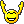SB Physics Help Room Sucks!
Would you like to react to this message? Create an account in a few clicks or log in to continue.

# Ch 22-2 Quiz##Ch 22-2 Quiz

Post help for the 22-2 quiz here

Question 1:
A glass lens with index of refraction 1.308 has a CONVEX surface with a radius of curvature of 14.42 cm and the other surface CONVEX too with a radius of curvature of 21.78 cm. What is the focal length in cm (see sheet 16,19) ? Indicate with a positive (negative) sign whether the lens is converging (diverging).

This is pretty easy, there is no converting and it is pretty much just plug-in.
Use the formula:
(1/f) = (n-1)(1/R1+1/R2)
Where you are solving for f
n = 1.308
R1 = 14.42 cm
R2 = 21.78 cm

Question 2:
Question 2: (1 point)

A glass lens with index of refraction 1.579 has a CONCAVE surface with a radius of curvature of 14.64 cm and the other surface CONVEX with a radius of curvature of 35.53 cm. What is the focal length in cm ? Indicate with a positive (negative) sign whether the lens is converging (diverging) (sheet 16,18,19).

This may appear to be the same as the first question but if you read more carefully it is concave for the first surface and convex for the second surface. Once this has be recognized, this problem is also very simple.

(1/f) = (n-1)(1/R1-1/R2) -> notice the only difference is that the fractions of the radii are being subtracted instead of added. Just plug in everything like before and you will get your answer. Once again, there is no converting or anything

Question 3: - another easy question. You guys probably dont even need help with this its so simple and all plug in but whatever

Question 3: (1 point)

An object is placed 35.75 cm from the midplane of a converging lens with a focal length of 12.59 cm. How far (meters) is the image from the midplane of the lens (see sheet 20') ? Indicate with a positive (negative) sign whether the image is inverted and real (upright and virtual). A useful cross check is the graphical method to find the image.

Use the equation (1/i) = (1/f)-(1/o)
You are given f and o, leave in centimeters when initially applying the equation, and once you solve for i convert to meters.

Question 4
A 1.482 mm high object is placed 2.847 cm from the midplane of a converging lens with a focal length of 7.831 cm. How high is the image in mm (sheet 20',20",22) ? Indicate with a positive (negative) sign whether the image is upright and virtual (inverted and real). A useful cross check is the graphical method to find the image

I'll do this one mathematically and show the work because it may be a bit harder to follow
First, determine what i is
(1/i) = (1/f)-(1/o)
(1/i)=(1/7.831)-(1/2.847)
(1/i) = -.2235
i = -4.473 cm = -.0447 m

Next, set up a ratio
Since m = (h_i/h_o) AND m = -(i/o), you can simply do (h_i/h_o) = -(i/o), where h_i is the height of the image and h_o is the height of the object
For this part I converted everything into meters
(h_i/.001482) = - (-.0447/.02847)
h_i/.001482 = 1.47
h_i = .002326 m = 2.23 mm

Kathleen

Posts : 42
Join date : 2008-12-18

##yeah

YOU ARE THE BEST AND I HOPE YOU GET INTO MED SCHOOL BEFORE ALL OF US!!!

yeah
Guest

##Go Kathleen

She probably will (get into med school first). If she's taking the time to understand and work through the problems,
and you are just plugging into equations as fast as you can, she's certainly going to do better on the course exams,
MCAT's, and probably even the exams you'll face in medical school.

Peter St
Guest

##Re: Ch 22-2 Quiz

Sadly to say guys, I'm better with the math then I am with the actual physics. I can study for hours and I still do average/below on the midterms. Same thing happened last semester. I'm just another one of those biology kids taking this because I have to =/ .

Kathleen

Posts : 42
Join date : 2008-12-18

##GO KAth

Hey u still rock who cares lolmoney23
Guest

##Re: Ch 22-2 Quiz